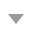OJ 同时被 3 个专栏收录67 篇文章 0 订阅

#### 问题描述

  template<class ElemType>

void Purge_Sq_OL( vector<ElemType> &L )；


（输入与输出之间用空行分隔）

#### 输入范例

8
2 3 3 5 5 5 7 11


#### 输出范例

2 3 3 5 5 5 7 11

2 3 5 7 11


#### 问题和解答• 个人以为是没有提供字符型测试案例，将main函数中地测试用例改成string类型后，发现仍旧不行。• 对比上面个两个图片，很容易发现如下两种情况

• 如果是字符型参加测试，4561没有办法通过测试
• 如果是整型参加测试，4608没有办法通过测试
• 如何才能同时识别两种数据？不好意思，想破脑袋都想不出来！

• 花了一分买了样例，4561• 又花了一分买样例，4608• 默默说一句，这个是真的。。。。。。

• 既然已经知道了所有的样例，后面就好做了，以string为基础喽，string就按照string做；int就将string转成int#### 分析与总结

• 不同的数据类型的比较方法是不同的，就拿11和8比较：

• 如果是按照字符串型进行比较的，8>11。字符串是将自己拆为字符型数据一个一个进行比较的。
• 如果是按照整型进行比较的，8<11
• 如下的删除模式是不对的。你删除一个元素之后，后面的元素会自动地向前移动，索引又自动加一，相当于中间就错过了一个元素。#### 代码实现——兄弟都是VIP了，那就改一下，不然重复率太高了，咱俩都得重写，不好

#include <iostream>
#include <vector>

using namespace std;

/*
description:function to compare two elements
*/
template<class Elemtype>
void sort(vector<Elemtype> &A)
{
Elemtype temp;
for(int i = 0;i < A.size();i ++)
{
for(int j = i + 1;j < A.size();j ++)
{
if(A.at(i) > A.at(j))
{
temp = A.at(i);
A.at(i) = A.at(j);
A.at(j) = temp;
}
}
}
}

/*
description:show all the elements of the vector
*/
template<class Elemtype>
void show(vector<Elemtype>& A)
{
typename std::vector<Elemtype> test = A;
typename std::vector<Elemtype>::iterator iter;
for(iter = test.begin();iter != test.end();iter ++)
{
cout<<*iter<<" ";
}
cout<<endl;
}

/*
description:
*/
template<class Elemtype>
void Purge_Sq(vector<Elemtype> &L)
{
if(L.empty())
{
show(L);
return;
}
sort(L);
show(L);

for(int i = 0 ;i < L.size() - 1;i ++)
{
for(int j = i + 1;j < L.size(); j++)
{
if(L.at(i) == L.at(j))
{
L.erase(L.begin() + j);
j --;
/*when the vector erase the element ,
the following part of the deleted one will automatically move forward
dring the time the index of i puls one
which leading jump over two one element!
*/
}
else
{
break;
}
}
}
cout<<endl;
show(L);
}

int main()
{
int Asize;
cin>>Asize;
string str;
cin>>str;
if(str == "a")
{
vector<string> A(Asize);
A.at(0) = str;
for(int i = 1 ; i < Asize; i ++) {
cin>>str;
A.at(i) = str;
}
Purge_Sq(A);
}
else
{
vector<int> B(Asize);
B.at(0) = atoi(str.c_str());
int temp;
for(int i = 1 ; i < Asize; i ++) {
cin>>temp;
B.at(i) = temp;
}
Purge_Sq(B);
}
return 0;
}• 函数构成的伪码(学习伪码的书写)
• 获取B中的每一个元素
• 调用函数：GetElem（L，i，&e），用e返回线性表L中的索引为i的元素
• 判定获取到的元素在新的线性表中是否存在
• 调用函数：LocateElem（L，e，compare()）,返回L中第一个与e，满足compare（）关系的元素的索引，没有就返回空
• 如果不存在，就将该元素加入新的线性表，存在就跳过
• 调用函数：ListInsert（&L，i，e），在线性表L中索引为i的位置插入元素e，并将线性表的长度加1
/*
description:construct a new list without any repeating element
*/
void pruge(List& A,List&B)
{
InitList(A);
La_len = 0;
//创建一个索引，用来记录当前的位置
Lb_len = ListLength(LB);
//创一个索引，用来遍历的对应的元素
for(i = 1;i <= Lb_len;i ++)
{
GetElem(LB,i,e);
//将LB中索引为i的元素，赋值给元素e
if(!LocateElem(LA,e,equal()))
//判定在LA中，是否有于元素e相等的元素
{
ListInsert(LA,++La_len,e);
//在LA的末尾，即La_lne处插入对应的元素e
}
}
}

{

03-0416812-026001
10-18543
10-18274
02-05576
10-0642
09-23
10-07333
10-06796
06-111万+点击重新获取扫码支付余额充值